Mobile QR Code1. (1 Department of Electronics & Communication Engineering, J. C. Bose University of Science & Technology, YMCA, Faridabad-121006, India )
2. ( Department of Electronics & Communication Engineering, Amity University Haryana, Gurugram-122413, India)

Channel engineering, Gate stack engineering, High- κ materials, Short channel effects, MOSFETs.

## I. INTRODUCTION

The mobile communication and telecom sectors are the fastest growing fields. The customer demands high speed, small size, and low power consumption devices. But conventional MOSFET has reached its scaling limit due to existence of various short channel effects (SCEs) (1). A new method must be found out to satisfy the high demands in the electronics world. Finally, a viable solution has emerged, with gate stack and channel engineering in the DH-DD-TM-SG MOSFET. This development in MOSFET has become the most suitable choice for the next generation devices. But, carry new challenges and opportunities for manufacturing and design. Gate stack structure is scrutinized with high dielectric materials to diminish leakage current (2-3). The Hafnium oxide is proposed as an alternate choice for gate oxide owing to its better thermal stability. Gate stack have equivalent oxide thickness (EOT) of 1.78 nm with insignificant leakage through gate oxide (4). Researchers have advised numerous high-κ dielectric materials for gate oxide which consists of hafnium-based oxide and aluminum-based oxides (5). The basic properties of good dielectrics are insulation and formation of capacitance. The band offset value should be more than 1ev to reduce the carrier injections in the bands. Good Thermal stability and high re- crystallization temperature should also possess by the oxide materials (6-8). The density of interface trap charges diminishes with a narrow interfacial oxide layer in gate stack. The field inside the channel boosts up and significant reduction in leakage current is observed in gate stack (9). Depending upon all these requirements, five dielectric materials are considered for the present investigation. In the literature, the suitable range of band gap is reported as 5.16 to 7.8 eV (10-11). The properties of various high-κ dielectric materials are reported by Nirmal et al. (12). The band gap of silicon oxide is very high and for titanium oxide is very low in contrast to requirements. So, hafnium oxide is suitable material for gate stack structure along with silicon oxide. It shows higher degree of potential at higher temperature condition for MOSFET.

The manufacturing viability of proposed device using different approaches is available in literature. The triple metal has been fabricated by utilizing Molybdenum (Mo) acting as gate material as its work function can be change by varying N$_{2}$ implant. Cylindrical gate stack source and drain is formed by using deep trench etching. Dual dielectric is formed by deposition of oxide layers. Similar types of devices were fabricated in the literature. However, fabrication of proposed structure has not yet done (13-16).

DH-DD-TM-SG MOSFET has been promising device for mixed signal applications due to cutback in SCEs and leakage current. Distinct high dielectric constant materials are utilized in the device and comparison has been carried out among their performances. Short channel behavior of proposed device has been investigated and proposed device reveals outperform performance. Atlas device simulator is used for the simulation. The proposed device is designed and simulated to find out various short channel performance metrics. The effect of high-κ dielectric is examined by substituting the oxide in the gate stack. The hafnium oxide shows an excellent performance with amended transconductance and drain current.

## II. ANALYTICAL MODEL

Fig. 1(a) & 1(b) depicts the exploded diagram of DH-DD-TM-SG MOSFET and simulated structure in device simulator. Channel engineering is incorporated at gate electrode using three different gate materials with changed work function of metals. The work function of metals are $\phi _{M1}$= 4.8 eV (Au), $\phi _{M2}$= 4.6 eV (Mo) and $\phi _{M3}$= 4.4 eV (Ti) respectively. It improves the carrier transportation efficiency and current. If order of metal work functions is changed then impact ionization occurs and device performance degrades (17-18).

Gate stack consist of two dielectrics which improves the sub-threshold behavior of the device. The symmetric dual halo doping is integrated into the structure which reduces the SCEs.

### (1) Surface Potential

The electrostatic potential formed by surface confined charges is known as the surface potential. The Poisson’s equation is used to determine the potential in the channel. It is given as:

##### (1)
$\frac{1}{r}\frac{\partial }{\partial r}\left(r\frac{\partial \left[\phi _{p}(r,z)\right]}{\partial r}\right)+\frac{\partial ^{{^{2}}}\left[\phi _{p}(r,z)\right]}{\partial z^{2}}=\frac{qN_{ap}}{\varepsilon _{Si}}$($L_{p- 1}\leq z\leq L_{p}$)

Where p=1, 2, 3, 4, 5

The parabolic approximation is used to calculate the potential distribution and is given by (19).

##### (2)
\begin{equation} \phi \left(r,z\right)=\chi _{0}\left(z\right)+\chi _{1}\left(z\right)\mathrm{r}+\chi _{2}\left(z\right)\mathrm{r}^{2} \end{equation}

Where the value of constant$\chi _{0}(z)$,$\chi _{1}(z)$ and $\chi _{2}(z)$can be achieved by using boundary conditions given by (9). Potential is given as

##### (3)
$\frac{d^{2}\phi _{sp}(z)}{dz^{2}}- \phi _{sp}(z)\left(\frac{2C_{{_{oxdh}}}}{\varepsilon _{Si}R}\right)+(VGS- V_{fbp})\left(\frac{2C_{{_{oxdh}}}}{\varepsilon _{Si}R}\right)=\frac{qN_{ap}}{\varepsilon _{Si}}$

$C_{{_{oxdh}}}$ is the gate oxide capacitance.

##### (4)
\begin{equation} C_{{_{oxdh}}}=\frac{\varepsilon _{Si{O_{2}}}}{R\ln \left[1+\frac{t_{oxdh}}{R}\right]} \end{equation}

Where $t_{oxdh}$ is the gate oxide thickness (20).

##### (5)
\begin{equation} t_{oxdh}=t_{Si{O_{2}}}+\frac{\varepsilon _{Si{O_{2}}}}{\varepsilon _{High- \kappa }}t_{High- \kappa } \end{equation}

Value of $\varepsilon _{High- \kappa }$ is changes according to dielectric materials. The equation (3) Can be written as:

##### (6)
\begin{equation} \frac{d^{2}\phi _{sp}\left(z\right)}{dz^{2}}- \theta ^{2}\phi _{sp}\left(z\right)=\chi _{p} \end{equation}

The solution of equation (6) is given as

##### (7)
\begin{align} \begin{array}{l} \phi _{sp}\left(z\right)=\alpha _{p}e^{\left(\theta z\right)}+\beta _{p}e^{\left(- \theta z\right)}- \frac{\chi _{p}}{\theta ^{2}}\\ \end{array} \end{align}

Where $\theta ^{2}=\frac{2C_{{_{oxdh}}}}{\varepsilon _{Si}R}$

\begin{equation*} \chi _{p}=\frac{qN_{ap}}{\varepsilon _{Si}}- \theta ^{2}\left(VGS- \mathrm{v}_{fbp}\right) \end{equation*}

Where $\alpha _{p}$ & $\beta _{p}$ are arbitrary constants, continuity equations for the potential ($\phi$) and field (E) are used to find out value of constants (9).

### (2) Electric Field

The electric field is extracted by differentiating potential w.r.t. z.

##### (8)
$E_{\mathrm{p}(z)}=- \frac{d\phi _{sp}(z)}{dz}=\theta (\beta _{p}e^{\left(- \theta z\right)}- \alpha _{p}e^{\left(\theta z\right)})$

### (3) Threshold Voltage

The device turns ON voltage is threshold voltage which is twice the Fermi potential and equal to the minimum surface potential.

##### (9)
$$\phi_{\min s p}=2 \phi_{f}$$

##### (10)
\begin{align} \begin{array}{l} 2\sqrt{\alpha _{1}\beta _{1}}- \frac{\chi _{1}}{\theta ^{2}}=2\frac{KT}{q}\left.\ln \left(\frac{N_{ap}}{n_{i}}\right)\right| _{VGS={v_{th}}}\\ \end{array} \end{align}

At the location of $\phi _{minsp}$, the sub-threshold leakage current starts in the device. So, it is very useful for modeling of the threshold voltage.

## III. RESULTS & DISCUSSION

The device parameters used during simulation are listed in Table 1.

Table 1. summarizes the device parameters used for simulation

 Parameters DH-DD-TM-SG S/D Doping (/m$^{3}$) 1x10$^{26}$ Channel Doping (/m$^{3}$) 1x10$^{23}$ Halo Doping (/m$^{3}$) 1x10$^{24}$ T$_{\mathrm{ox}}$ (nm) 1.78 T$_{\mathrm{Si}}$ (nm) 10 Channel Length (nm) 30 Permittivity of SiO$_{2}$ 3.9 Permittivity of Al$_{2}$O$_{3}$ 8 Permittivity of LaAlO$_{3}$ 15 Permittivity of HfO$_{2}$ 21 Permittivity of TiO$_{2}$ 40 VGS 0.2 V VDS 0.1 V

The simulated data of proposed model is calibrated with the simulated work (21) which validates the models used for the device simulation. Fig. 2 depicts that simulated results are well matched with the experimental results.

Fig. 2. Calibration of proposed device results with experimental results.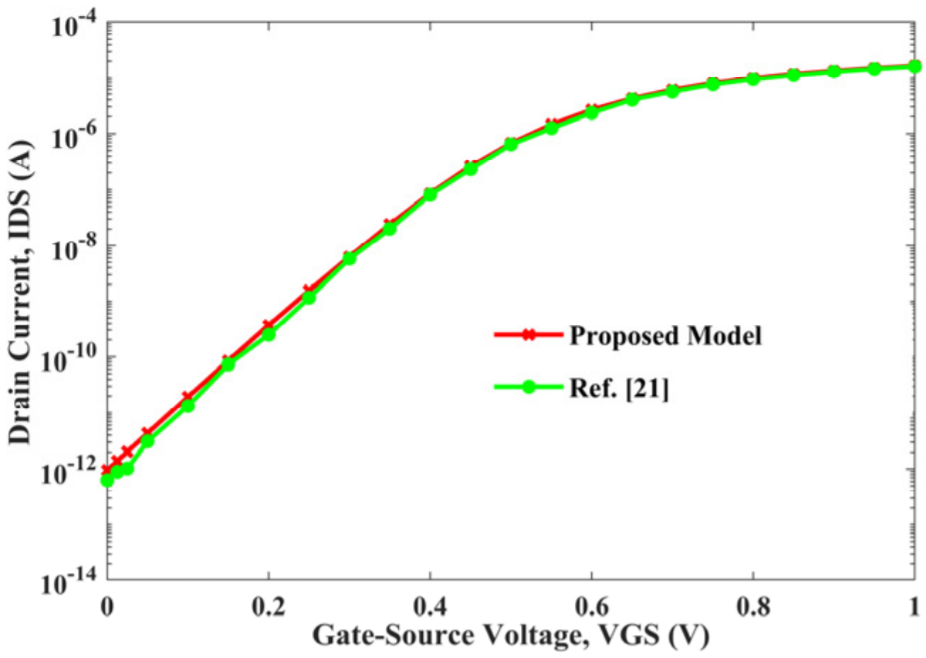### 1. Surface Potential

The present analysis is carried out for surface potential$\phi _{sp}$. The different metal work function produces step potential profile which minimizes the SCEs and screening of a channel province under high metal work function from the variation in the drain potential. The lower metal gate work function near to drain side absorbs the extra drain bias variation which mitigates the DIBL (22-23). The difference in work function among the interfaces of metals creates the step up in the potential profile. Fig. 3 shows that triple material structure has two step function profile which is a clear indication of a reduction in SCEs. These gradual steps function profile at the interface screens the higher metal gate M$_{1}$ work function region from the fluctuation of drain potential. Enhanced VDS is discarded across the lower metal gate M$_{3}$ work function region. It is noticed that the minimum surface potential $\phi _{sp}$ happens for DH-DD-TM-SG MOSFET in the halo region. In this novel device, there are additional steps at the drain and source sides. Normally, there are two step function profiles in TM-SG but for dual halo, it is realized that the surface potential of DH-DD-TM-SG exhibits four step function profiles. So, this extra step profile further helps in scale down the short channel influence and improving the current driving capability. The analytical results are well in agreement with simulated results validating the model (24). Fig. 3 highlights the potential of proposed device with different values of ${k}$. The step rise in potential is observed in halo doped region of 1.05 V as compared to the rest of the portion which shows potential of 0.78 V which is due to sudden change in doping. The value of surface potential is more for Al$_{2}$O$_{3}$ as compared to other dielectrics due to its lower physical thickness. TCAD Silvaco is used for extracting the simulation data and MATLAB is used for plotting and solving mathematical equations (25).

Fig. 3. Potential of proposed device with various dielectric materials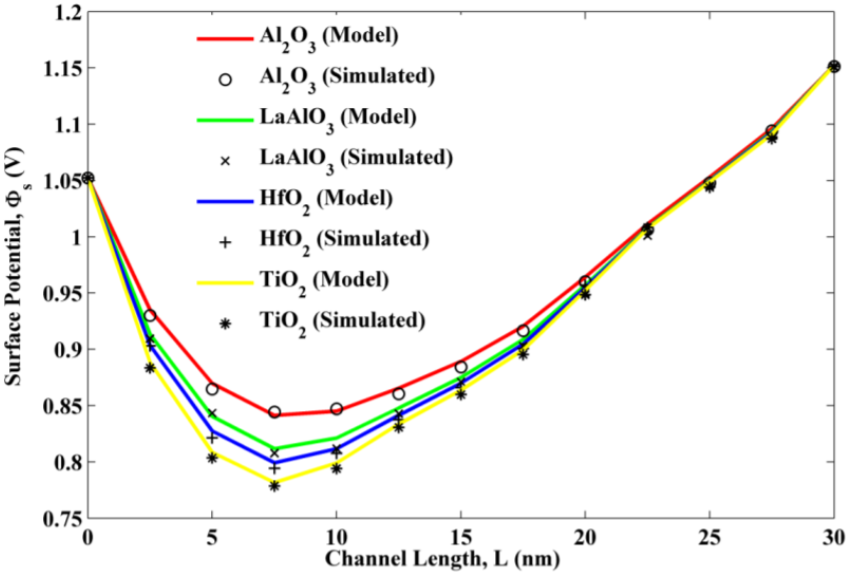### 2. Electric Field

Fig. 4. Field of proposed device with various dielectric materials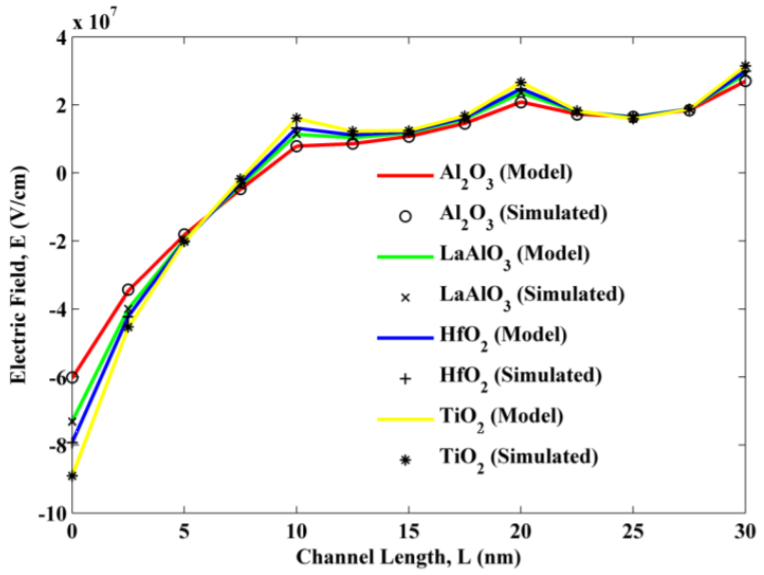Fig. 4 reveals the field of DH-DD-TM-SG MOSFET with various dielectric constants. It is also observed from the figure that the increase in $k$ values enhances the field in the channel. The extra peak of field is detected at the interfaces of metals. These peaks decrease the field at drain terminal which causes diminution in DIBL and hot carrier effects. These are the prime SCEs in MOSFET which reduces its performance at lower dimension.

### 3. Threshold Voltage

Fig. 5 shows the threshold voltage of DH-DD-TM-SG MOSFET. It is also observed from the figure that the increase in $k$ values enhances the threshold voltage. Lower value of threshold voltage of a device increases the leakage current and higher value of threshold voltage reduces the operation speed of a device. The proposed device has moderate value of threshold voltage as compared to its counterpart. It indicates improvement in gate controllability due to channel engineering. Hence, proposed device is worthy for low power applications.

Fig. 5. Threshold voltage of proposed device with various dielectric materials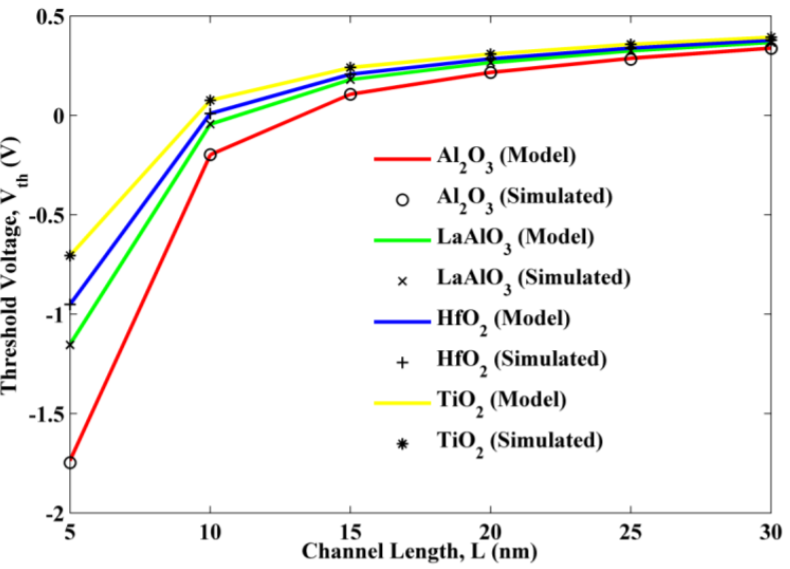### 4. Sub-threshold Current

Fig. 6. Sub-threshold current of proposed device with various dielectric materials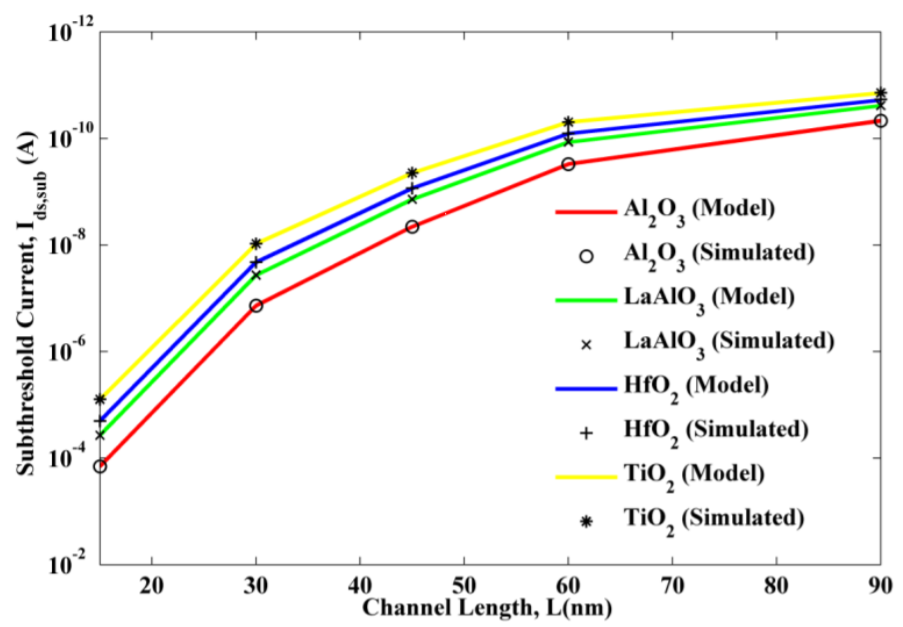Fig. 6 depicts the sub-threshold current with various dielectric constant. The leakage current of device with LaAlO$_{3 }$as dielectric is 0.369X10$^{-9}$ A. ID$_{\mathrm{, sub}}$ of HfO$_{2}$ is 0.210X10$^{-9}$ A, which indicate significant reduction in leakage current of 42.94% as compared to others. It is noticed from the Fig. 6 that leakage current reduces exponentially with increase in dielectric value. The high- $k$ materials provide more physical thickness which diminishes the tunneling of carriers through the insulator. So, proposed device with HfO$_{2}$ as dielectric significantly mitigates the leakage current which makes device suitable for low power applications.

### 5. DIBL Effect

A large bias at the drain causes DIBL in the MOSFET which degrade the device performance. The barrier height between source and channel becomes lowered (26). Thus, control of gate terminal decreases over the channel. Fig. 7 illustrates the DIBL with varying dielectric constant. It is observed from the figure that SiO$_{2}$ has highest value of DIBL which is 9.49 mV/V. As the value of $k$ increases then corresponding exponentially decrement in DIBL are observed. HfO$_{2}$ reveals DIBL of 0.88 mV/V which points out significant reduction in DIBL. When DIBL of proposed device is compared with DIBL of Nirmal et al. (12) device it shows improvement of 26 %. Hence, proposed device provides better reduction of SCEs.

Fig. 7. DIBL effect of DH-DD-TM-SG MOSFET with various dielectric materials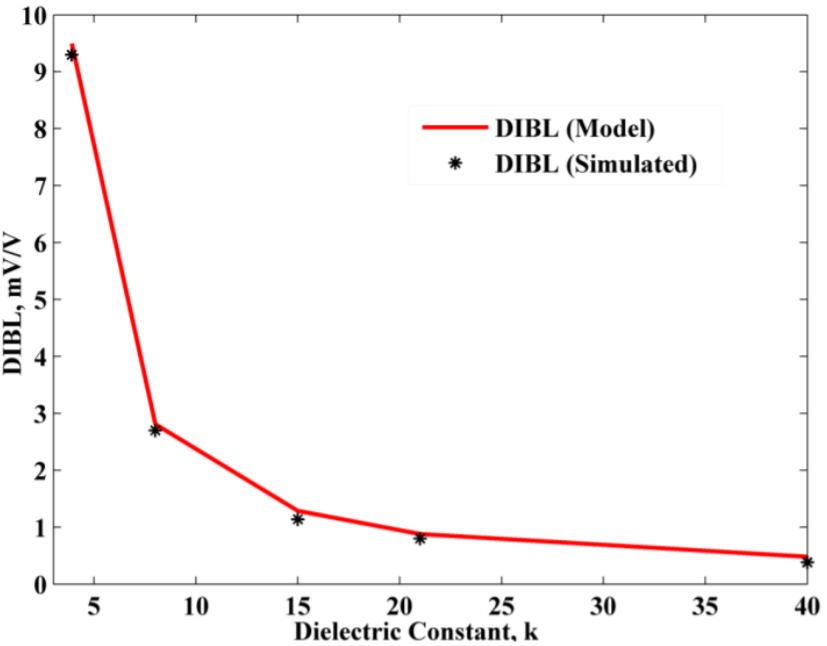### 6. Threshold Voltage Roll-off Effect

The attenuation of threshold voltage with attenuation in channel length is called “V$_{\mathrm{th}}$-roll off” (27-28). Fig. 8 depicts the roll-off of threshold voltage. The V$_{\mathrm{th}}$-roll off has been calculated by finding the deviation in threshold voltage for small and long channel devices. It is observed from the figure that SiO$_{2}$ has highest value of V$_{\mathrm{throll}}$ which is -0.15 V. As the value of $k$ increases then corresponding exponentially increase in V$_{\mathrm{throll}}$ are observed. HfO$_{2}$ reveals V$_{\mathrm{throll}}$ of -0.09 V which points out significant reduction in V$_{\mathrm{throll}}$.

Fig. 8. Threshold voltage roll-off of DH-DD-TM-SG MOSFET with various dielectric materials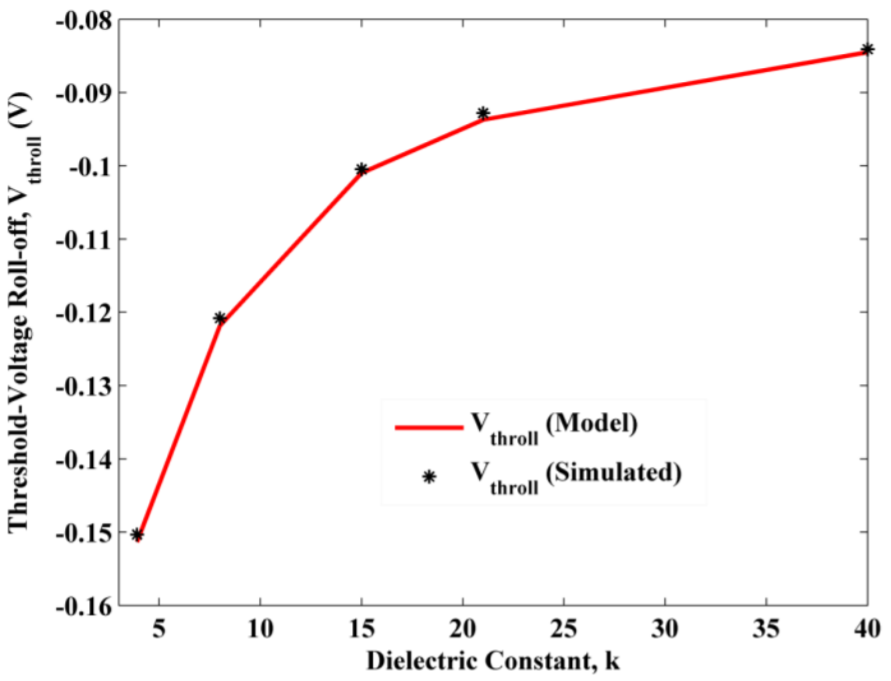### 7. Sub-threshold Swing

SS is the minimum voltage required to enter into the ON state from the OFF state. The ideal value of SS is 60 mV/decade. Fig. 9 shows the SS as a function of variation in dielectric constant. SS decrease with increase in value of $k$. It is observed from the figure that HfO$_{2}$ shows an improvement of 15.34% as compared to SiO$_{2}$ due to reduce leakage current in former oxide.

Fig. 9. Sub-threshold swing of proposed device with various dielectric materials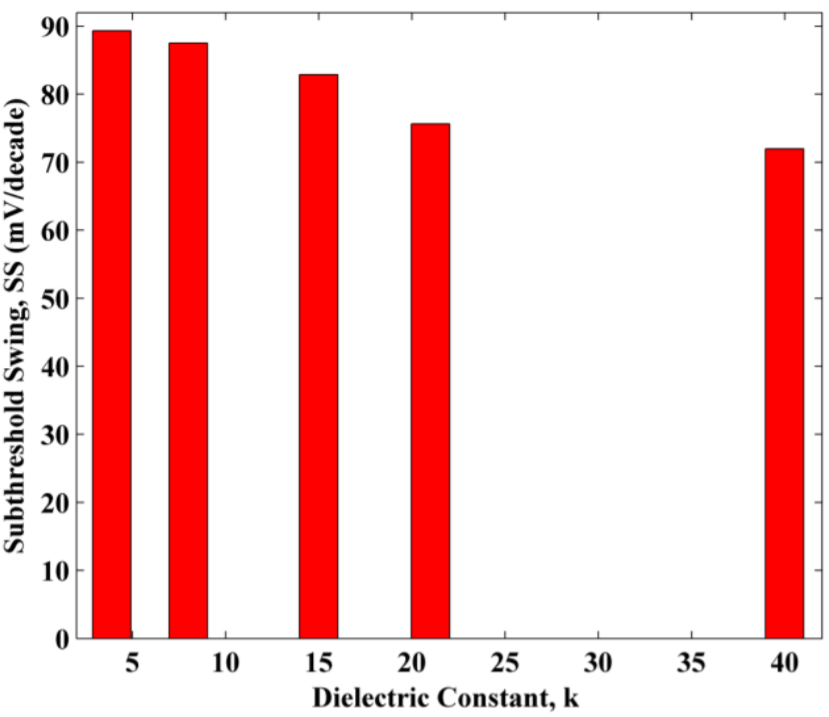## IV. CONCLUSIONS

A 2D analytical model of proposed device has been presented for various gate dielectrics in terms of potential, field, and threshold voltage. This paper also described the various SCEs like DIBL, V$_{\mathrm{throll}}$ and SS. HfO$_{2 }$dielectric shows an excellent immunity against SCEs as compared to its counterpart. Potential and field exhibit extra peaks in their responses which is a clear sign of mitigation in HCEs. The improvement in DIBL, V$_{\mathrm{throll}}$ and SS are the direct consequences of gate stack and channel engineering. Leakage current is a key metric for standby power dissipation in device. HfO$_{2}$ exhibits very less leakage current as compared to SiO$_{2}$. Hence, proposed device is very useful for low power applications. In future, HfO$_{2 }$becomes the popular choice as a good insulator in the new generation devices.

### REFERENCES

1
Nirmal D., 2013, Subthreshold analysis of nanoscale FinFETs for ultra low power application using high-k materials, Electronics, International Journal of, Vol. 100, No. 6, pp. 803-8172
Ghosh P., 2012, An Accurate Small Signal Modeling of Cylindrical/Surrounded Gate MOSFET for High Frequency Applications, Semiconductor Technology and Science, Journal of, Vol. 12, No. 4, pp. 377-3873
Ghosh P., 2012, An Analytical Drain Current Model for Dual Material Engineered Cylindrical/Surrounded Gate MOSFET, Microelectronics Journal, Vol. 43, No. 1, pp. 17-244
Gupta N., March, 2018, Modeling and analysis of Threshold Voltage for Dual-Halo Dual-Dielectric Triple-Material Surrounding-Gate Metal–Oxide–Semiconductor Field-Effect Transistors, Pure and Applied Mathematics, International Journal of, Vol. 118, No. 18, pp. 3759-37715
Robertson J., 2004, High dielectric constant oxides, Applied Physics, The European Physical Journal, Vol. 28, No. 3, pp. 265-2916
Huang A.P., 2010, Hafnium-based High-k Gate Dielectrics, Advances in Solid State Circuits Technologies, pp. 333-3507
Wilk G.D., 2001, High-k gate dielectrics: Current status and materials properties considerations, Applied Physics, Journal of, Vol. 89, No. 10, pp. 5243-52758
Manoj C.R., 2007, Impact of High-k gate dielectrics on the device and circuit performance of Nanoscale FinFETs, Electron Device Letters, IEEE, Vol. 28, No. 4, pp. 295-2979
Gupta N., Nov, 2018, Performance and a new 2-D analytical modeling of a Dual-Halo Dual-Dielectric Triple-Material Surrounding-Gate-All-Around (DH-DD-TM-SGAA) MOSFET, Engineering Science and Technology, Journal of, Vol. 13, No. 11, pp. 3619-363110
Nirmal D., 2010, Nanosized High k Dielectric Material for FINFET, Integrated Ferroelectrics, Vol. 121, No. 1, pp. 31-3511
Rollo S., 2020, High performance Fin-FET electrochemical sensor with high-k dielectric materials, In: Sensors and Actuators, B: Chemical, Vol. 303, pp. 1-712
Nirmal D., 2012, Nanoscale channel engineered double gate MOSFET for mixed signal applications using high-k dielectric, Circuit Theory and Applications, International Journal of, Vol. 41, No. 6, pp. 608-61813
Tekleab D., Dual high-k oxides with SiGe channel, NY (US), US 2011/8017469B214
Tekleab D., Silicon nanotube MOSFET, NY (US), US 2014/8871576B215
Yin C., 2003, Fabrication of Raised S/D Gate-All-Around Transistor and Gate Misalignment Analysis, IEEE Electron Device Letters, Vol. 24, No. 10, pp. 658-66016
Lin R., 2002, An adjustable work function technology using Mo gate for CMOS devices, IEEE Electron Device Letters, Vol. 23, pp. 49-5117
Meiwes-Broer K.H., 1994, Work functions of metal clusters, Hyperfine Interact, Vol. 89, pp. 263-26918
Ghosh P., 2012, ,Analytical modeling and simulation for dual metal gate stack architecture cylindrical/surrounded gate MOSFET, Journal of Semiconductor Technology and Science, Vol. 12, No. 4, pp. 458-46319
Young K. K., 1989, Short channel effect in fully depleted SOI MOSFETs, Electron Device IEEE Transactions on, Vol. 36, No. 2, pp. 399-40220
Gupta N., July 2018, An accurate 2D Analytical Model for Transconductance-to-Drain Current ratio (gm/Id) for a Dual-Halo Dual-Dielectric Triple-Material Cylindrical-Gate–All-Around (DH-DD-TM-CGAA) MOSFETs, Engineering International Journal of, Vol. 31, No. 07, pp. 1038-104321
Vanitha P., et al , 2015, Triple Material Surrounding Gate (TMSG) Nanoscale Tunnel FET-Analytical Modeling and Simulation, Journal of Semiconductor Technology and Science, Vol. 15, No. 6, pp. 585-59322
Dhanaselvam P. S., et al , 2014, A 2D analytical modeling of single halo triple material surrounding gate MOSFET, Electrical Engineering & Technology Journal of, Vol. 9, No. 4, pp. 1355-135923
Venkatesh M., 2020, Influence of Threshold Voltage Performance Analysis on Dual Halo Gate Stacked Triple Material Dual Gate TFET for Ultra Low Power Applications, Silicon, pp. 1-1324
Rewari S., 2015, Numerical modeling of subthreshold region of junctionless double surrounding gate MOSFET, Superlattices and Microstructures, Vol. 90, pp. 8-1925
ATLAS 3D DEVICE Simulator , 2010, SILVACO International26
Cho H., 2019, DIBL enhancement in ferroelectric-gated FinFET, Semiconductor Science and Technology, Vol. 34, No. 2, pp. 1-527
Islam Md. S., 2020, HfO2/TiO2/HfO2 tri-layer high-K gate oxide based MoS2 negative capacitance FET with steep subthreshold swing, American Institute of Physics, Vol. 10, pp. 035202:1-828
Dhanaselvam P. S., et al , 2013, Analytical approach of a nanoscale triple material surrounding gate (TMSG) MOSFETs for reduced short-channel effects, Microelectronics Journal, Vol. 44, No. 5, pp. 400-404## Author

Prashant Kumar received his M.Tech. degree in VLSI Design & Embedded System from G.J.U. of Science & Technology, Hisar, India in 2008 and currently pursuing his Ph.D. in Electronics Engineering Department at J.C. Bose University of Science & Technology, YMCA, Faridabad. His interests include high speed CMOS circuit design and digital mixed-signal ICs, Semiconductor device modeling.

Neeraj Gupta was born in Karnal, India, on 1984.

He received the PhD degree in Engineering from Amity University Haryana, in 2019.

He is having more than 70 publications in various journals and conferences to his credits.

His interests include device modeling, digital VLSI design circuits and ultra-low-voltage analog circuits.

Nitin Sachdeva received her M.Tech. degree in VLSI Design and CAD from Thapar Institute of Engineering and Technology, Punjab, India in 2005 and currently pursuing his Ph.D. in Electronics Engineering Department at J.C. Bose University of Science & Technology, YMCA, Faridabad. Her interests include CMOS Digital design, Analog circuit design and Semiconductor device modeling.

Tarun Kumar Sachdeva received his M.Tech. Degree in VLSI Design and CAD from Thapar Institute of Engineering and Technology, Punjab, India in 2005 and currently pursuing his Ph.D. in Electronics Engineering Department at J.C. Bose University of Science & Technology, YMCA, Faridabad.

His interest is in area of Modeling and Simulation of Nanoscale Semiconductor Devices.

Munish Vashishath has done his Ph.D. in the domain of semiconductor device modeling.

He is having more than 150 publications in various journals and conferences to his credits.

He has guided 2 Ph.D. scholars and currently, 5 others are pursuing Ph.D. under him.

His research interest includes the device modeling and embedded system development.